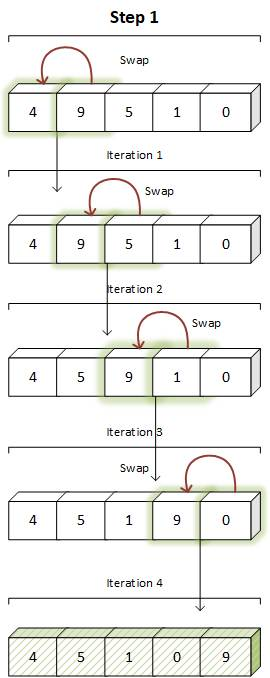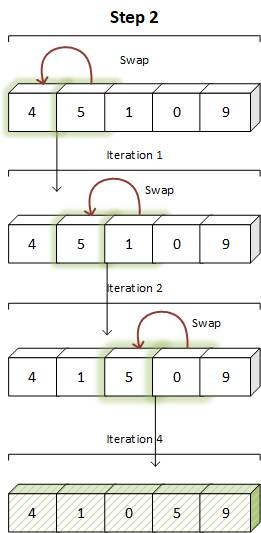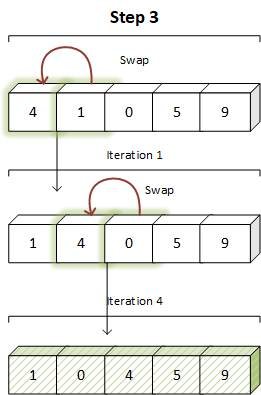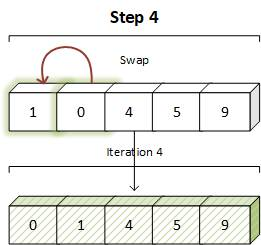# Array C Programing

Bubble Sort

In bubble sort, each element is compared with its adjacent element, if the first element is larger than the second one then the position of the elements are interchanged otherwise it is not changed. Then next element is compared with its adjacent element and the same process is repeated for all elements in the array.

During the next pass the same process is repeated leaving the largest element. During the pass, the second largest element occupies the second last position.

During the next pass, the same process is repeated leaving the largest element. During this pass, the largest element occupies the n-1 position. This process is repeated until no more elements are left for comparisons. Finally array is sorted one.

Algorithm :

1. Input n elements of an array a
2. Initialize i = 0
3. Repeat through step 6 while (i < n).
4. Set j = 0.
5. Repeat through step 6 while (j < n - i - 1)
6. if (a [j] > [j+1])
7. (a) temp = a [j],
8. (b) a [j] a[j + 1],
9. (c) a [j + 1] = temp.
10. Display the sorted elements of array a
11. Exit.

The following is a C program source code for a 5-integer sorting of Bubble. The code sorts the elements in ascending order.

``````

#include
void swap_node(int *x, int *y)
{
int tmp;
tmp = *x;
*x = *y;
*y = tmp;
}
void bubble_sort(int elements[], int count)
{
int step, index;
printf("Bubble sort started-\n");
for (step = 0; step < count - 1; step++)
{
printf("==== Step %d ====\n", step + 1);
print_array(elements, 5);
for (index = 0; index < (count - step - 1); index++)
{
printf("#%d ", index + 1);
if (elements[index] > elements[index + 1])
{
printf("   swap  %d >  %d\n",
elements[index],
elements[index + 1]);
swap_node(&elements[index],
&elements[index + 1]);
}
else
{
printf("no swap  %d <= %d\n",
elements[index],
elements[index + 1]);
}
print_array(elements, 5);
}
}
printf("Bubble sort ended\n");

}
void print_array(int array[], int count)
{
int i;
printf("[");
for (i = 0; i < count; i++)
printf("%d, ", array[i]);
printf("\b\b]\n");
}
int main(int argc, char *argv[])
{
int array = { 4, 9, 5, 1, 0 };
printf("Unsorted elements\n");
print_array(array, 5);
bubble_sort(array, 5);
printf("Bubble sorted elements\n");
print_array(array, 5);
}
``````

Output: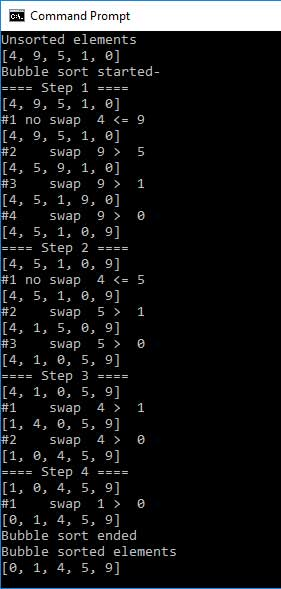Output: